# GATE’22 EE: Weekly Practice Quiz-01

Each question carries 2 marks.
Negative marking: 2/3 mark
Total Questions: 10
Time: 20 min.

Q1. For the circuit given in figure below the power delivered by the 2-volt source is given by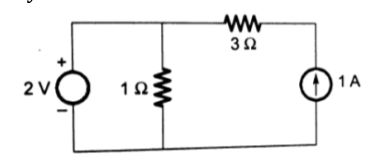(a) 4 W
(b) -4 W
(c) 2 W
(d) -2 W

Q2. If the torque/weight ratio of an instrument is low, then it can be concluded that
(a) The meter will have a uniform scale
(b) The meter will have the non-uniform scale
(c) The sensitivity of the meter will be high
(d) The sensitivity of the meter will be low

Q3. The Y_bus matrix of a 100-bus interconnected system is 80% sparse. Then, the number of transmission lines in the system must be
(a) 1500
(b) 1900
(c) 3000
(d) 950

Q4. The bus admittance matrix of a power system is given by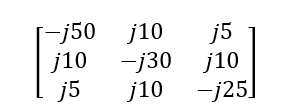The impedance of line between bus 2 and 3 will be equal to:
(a) -j0.5
(b) j0.1
(c) j0.2
(d) -j0.2

Q5. There are N resistances, each are connected in parallel having value R with the equivalent resistance of X. What will be the total resistance when these N resistances are connected in series?
(a) NX
(b) RNX
(c) X/N
(d) N2X

Q6. A chopper circuit fed from an input voltage of 20 V DC, delivers a load power of 16 W. for a chopper efficiency of 0.8, the input current is
(a) 0.8 A
(b) 0.64 A
(c) 1 A
(d) 1.25 A

Q7. The Fourier series of a real periodic function has only
(P) cosine terms if it is even
(Q) sine terms if it is even
(R) cosine terms if it is odd
(S) sine terms if it is odd
Which of the above statements are correct?
(a) P and S
(b) P and R
(c) Q and S
(d) Q and R

Q8. If, in a DC machine, the ratio of constant losses to armature resistance is 3, then at maximum efficiency, the magnitude of current flowing will be:
(a) 3 A
(b) 1.732 A
(c) 9 A
(d) 6.667 A

Q9. Which of the following features are offered by a bipolar junction transistor amplifier in Darlington connection?
1. High current gain
2. High voltage gain
3. High input impedance
Select the correct answer using the code given below:
(a) 1 and 2 only
(b) 2 and 3 only
(c) 1 and 3 only
(d) 1, 2 and 3

Q10. The currents in a 3-phase unbalanced system are:
(I_R ) =(12+j 6)A ;
(I_Y ) =(12-j 12)A ;
(I_B ) =(-15+j 10)A
The phase sequence is RYB. The zero-phase sequence component in R-phase is
(a) (1.5 + j 2.3) A
(b) (3 + j 1.33) A
(c) (2.8 + j 6.7) A
(d) (1.5 + j 0.15) A

SOLUTIONS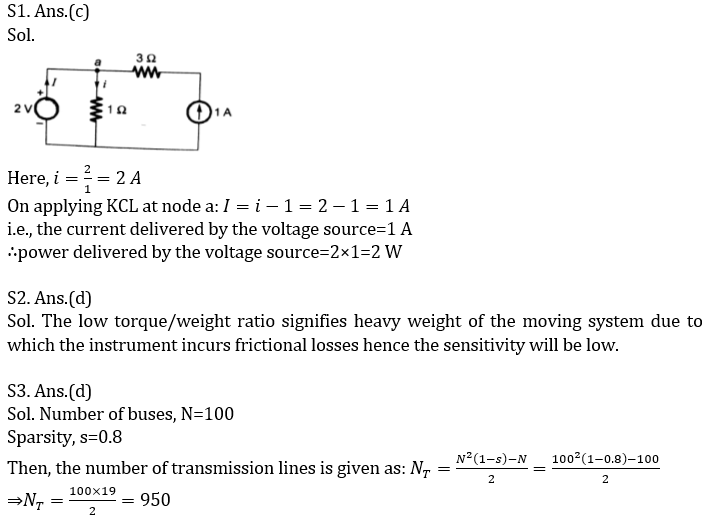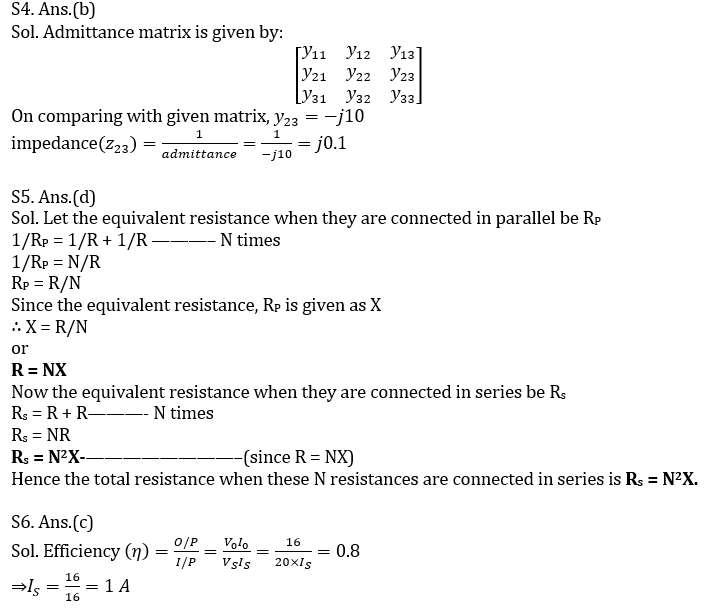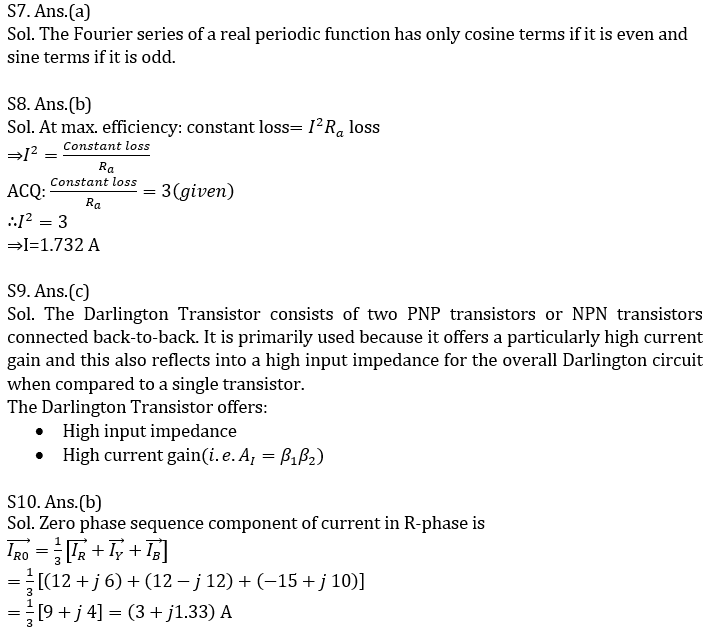×Join India's largest learning destination

What You Will get ?

•Job Alerts
•Daily Quizzes
•Subject-Wise Quizzes
•Current Affairs
•Previous year question papers
•Doubt Solving session

ORJoin India's largest learning destination

What You Will get ?

•Job Alerts
•Daily Quizzes
•Subject-Wise Quizzes
•Current Affairs
•Previous year question papers
•Doubt Solving session

ORJoin India's largest learning destination

What You Will get ?

•Job Alerts
•Daily Quizzes
•Subject-Wise Quizzes
•Current Affairs
•Previous year question papers
•Doubt Solving session

Enter the email address associated with your account, and we'll email you an OTP to verify it's you.Join India's largest learning destination

What You Will get ?

•Job Alerts
•Daily Quizzes
•Subject-Wise Quizzes
•Current Affairs
•Previous year question papers
•Doubt Solving session

Enter OTP

Please enter the OTP sent to
/6

Did not recive OTP?

Resend in 60sJoin India's largest learning destination

What You Will get ?

•Job Alerts
•Daily Quizzes
•Subject-Wise Quizzes
•Current Affairs
•Previous year question papers
•Doubt Solving sessionJoin India's largest learning destination

What You Will get ?

•Job Alerts
•Daily Quizzes
•Subject-Wise Quizzes
•Current Affairs
•Previous year question papers
•Doubt Solving session

Almost there

+91Join India's largest learning destination

What You Will get ?

•Job Alerts
•Daily Quizzes
•Subject-Wise Quizzes
•Current Affairs
•Previous year question papers
•Doubt Solving session

Enter OTP

Please enter the OTP sent to Edit Number

Did not recive OTP?

Resend 60

By skipping this step you will not recieve any free content avalaible on adda247, also you will miss onto notification and job alerts

Are you sure you want to skip this step?

By skipping this step you will not recieve any free content avalaible on adda247, also you will miss onto notification and job alerts

Are you sure you want to skip this step?